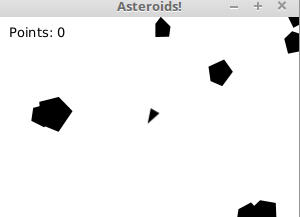Part 14

# Larger application: Asteroids

Asteroids developed by Atari and self published in the year 1979 is a video game classic. The gameplay consists of the player steering a triangular spaceship, with the goal of destroying asteroids by shooting them.

What follows is a larger scale example, where we create a part of the Asteroids game. The game is also an exercise in the course — write the game into the provided template (at the end of the example) by following the example.

The game is constructed in multiple parts, which are the following:

• Creating the game window
• Creating the ship
• Turning the ship
• Moving the ship
• Creating an asteroid
• The collision between the ship and an asteroid
• Multiple asteroids
• Staying within the window
• Projectiles

Let's begin making the application by creating the game window

## Creating the game window

We will build the application such that the game window may contain an arbitrary amount of elements, the positions of which will be ignored by the layout used. This task fits the Pane class. The Pane class contains a list of type ObservableList containing child elements. The list can be accessed using the `getChildren` method of the Pane class.

The program shown below creates a window that is 300 pixels wide and 200 pixels tall. At the point 30, 50 in the window is a circle with a radius of 10 pixels. In computer programs it is typical for the origin of the coordinate system is placed at the top left corner of the window. Additionally the value of the y-coordinate increases when moving down.

``````import javafx.application.Application;
import javafx.scene.Scene;
import javafx.scene.layout.Pane;
import javafx.scene.shape.Circle;
import javafx.stage.Stage;

public class PaneExample extends Application {

@Override
public void start(Stage stage) throws Exception {
Pane pane = new Pane();
pane.setPrefSize(300, 200);

Scene scene = new Scene(pane);
stage.setScene(scene);
stage.show();
}

public static void main(String[] args) {
launch(args);
}
}``````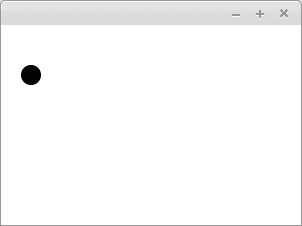We call our application `AsteroidsApplication`. AsteroidsApplication applies the above example. The application does not add a circle to the window, but we have provided a title for the application. The width of the window is 600 pixels and the height is 400 pixels.

``````import javafx.application.Application;
import javafx.scene.Scene;
import javafx.scene.layout.Pane;
import javafx.stage.Stage;

public class AsteroidsApplication extends Application {

@Override
public void start(Stage stage) throws Exception {
Pane pane = new Pane();
pane.setPrefSize(600, 400);

Scene scene = new Scene(pane);
stage.setTitle("Asteroids!");
stage.setScene(scene);
stage.show();
}

public static void main(String[] args) {
launch(args);
}
}``````

## Creating the ship

Next we create the ship. In Asteroids the ship is a triangle. The display of the triangle is possible using the Polygon class, which is used to represent polygons. The corners of the polygon are set for the Polygon object, either as parameters of the constructor or into the list contained within the Polygon class.

In the example below we have added a parallelogram that is 100 pixels wide and 50 pixels tall using the Polygon class.

``````@Override
public void start(Stage stage) throws Exception {
Pane pane = new Pane();
pane.setPrefSize(300, 200);

Polygon parallelogram = new Polygon(0, 0, 100, 0, 100, 50, 0, 50);

Scene scene = new Scene(pane);
stage.setScene(scene);
stage.show();
}``````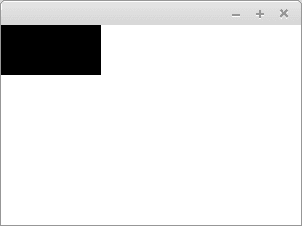Moving the polygon into a more fitting position can be done with the provided methods `setTranslateX` and `setTranslateY` of the Polygon class. In the example below we create a parallelogram just like before, but now the parallelogram has been moved 100 pixels to the right and 20 pixels down.

``````@Override
public void start(Stage stage) throws Exception {
Pane pane = new Pane();
pane.setPrefSize(300, 200);

Polygon parallelogram = new Polygon(0, 0, 100, 0, 100, 50, 0, 50);
parallelogram.setTranslateX(100);
parallelogram.setTranslateY(20);

Scene scene = new Scene(pane);
stage.setScene(scene);
stage.show();
}``````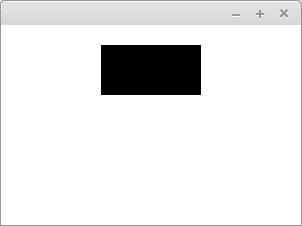Let's create a triangle representing the ship and add it to our AsteroidsApplication. We set the triangle at the center of the screen — because the width of the screen is 600 pixels and the height is 400 pixels, we move the triangle 300 pixels to the right and 200 pixels down.

``````import javafx.application.Application;
import javafx.scene.Scene;
import javafx.scene.layout.Pane;
import javafx.scene.shape.Polygon;
import javafx.stage.Stage;

public class AsteroidsApplication extends Application {

@Override
public void start(Stage stage) throws Exception {
Pane pane = new Pane();
pane.setPrefSize(600, 400);

Polygon ship = new Polygon(-5, -5, 10, 0, -5, 5);
ship.setTranslateX(300);
ship.setTranslateY(200);

Scene scene = new Scene(pane);
stage.setTitle("Asteroids!");
stage.setScene(scene);
stage.show();
}

public static void main(String[] args) {
launch(args);
}
}``````

## Turning the ship: Keyboard listener, part 1

Classes like Polygon and Circle inherit the Node class of JavaFx. The Node class has a variable `rotate`, which describes the rotation of the node in degrees. Turning any object inheriting the Node class is therefore quite straightforward — you just use the existing method `setRotate`. The method is given the amount to turn in degrees as its parameter.

In the example below we have modified a previous example such that the parallelogram is rotated 30 degrees.

``````@Override
public void start(Stage stage) throws Exception {
Pane pane = new Pane();
pane.setPrefSize(600, 400);

Polygon ship = new Polygon(-5, -5, 10, 0, -5, 5);
ship.setTranslateX(300);
ship.setTranslateY(200);
ship.setRotate(30);

Scene scene = new Scene(pane);
stage.setScene(scene);
stage.show();
}``````

In reality we don't want a situation where the ship only turns once, but one in which we can steer the ship while the game is running.

The `Scene` object describing the content of the window provides a method `setOnKeyPressed`, which can be given an object for handling events as its parameter. Let's create an event handler, which reacts to events on the keyboard. Keyboard events have an enumerated variable `KeyCode`, which tells us the key that was pressed. We are interested in the keys left (LEFT) and right (RIGHT).

First we make a test version, in which the turning of the ship is simple. If the user presses the left arrow, the degrees are set to -30. Then again, if the user presses the right key, then the degrees are set to 30.

``````scene.setOnKeyPressed(event -> {
if (event.getCode() == KeyCode.LEFT) {
ship.setRotate(-30);
}

if (event.getCode() == KeyCode.RIGHT) {
ship.setRotate(30);
}
});``````

If the ship was a parallelogram the functionality would look as follows: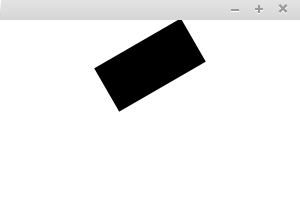The turning can be made smoother by utilizing existing information about the rotation. In the next example the ship turns five degrees at a time.

``````scene.setOnKeyPressed(event -> {
if (event.getCode() == KeyCode.LEFT) {
ship.setRotate(ship.getRotate() - 5);
}

if (event.getCode() == KeyCode.RIGHT) {
ship.setRotate(ship.getRotate() + 5);
}
});``````

Below is pictured an equivalent example, where instead of rotating the ship we rotate a parallelogram.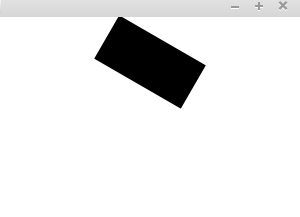## Turning the ship: Keyboard listener, part 2

The previous approach enables a "kind of OK"-way to turn a node. There is an issue with the approach — the movement is not smooth. When the key is pressed, the ship rotates, then takes a short break, after which it continues rotating.

This is related to how programs handle keyboard events by default. If the program would handle the keypress as multiple events immediately as the key is pressed, then, for example, writing text would become quite difficult, since even slightly longer keypresses would immediately generate multiple characters.

Let's change the handling of keyboard event, such that we keep a record of pressed keys. This can be done, for example, using a hash table. The hash table contains the KeyCode object, i.e. the object representing the key, as the key and a Boolean variable as the value. If the value of the boolean variable of a particular key is `true`, then the key is pressed, otherwise the key is not pressed.

Now we also consider depressing the key, i.e. the `onKeyReleased` event.

``````Map<KeyCode, Boolean> pressedKeys = new HashMap<>();

scene.setOnKeyPressed(event -> {
pressedKeys.put(event.getCode(), Boolean.TRUE);
});

scene.setOnKeyReleased(event -> {
pressedKeys.put(event.getCode(), Boolean.FALSE);
});``````

But! Nothing is currently turning the ship.

Indeed. We still need functionality for rotation. We will start using the AnimationTimer class, which is meant for creating animations, and assign it the responsibility of turning the the ship in case the left or right key is pressed.

``````Map<KeyCode, Boolean> pressedKeys = new HashMap<>();

scene.setOnKeyPressed(event -> {
pressedKeys.put(event.getCode(), Boolean.TRUE);
});

scene.setOnKeyReleased(event -> {
pressedKeys.put(event.getCode(), Boolean.FALSE);
});

new AnimationTimer() {

@Override
public void handle(long now) {
if(pressedKeys.getOrDefault(KeyCode.LEFT, false)) {
ship.setRotate(ship.getRotate() - 5);
}

if(pressedKeys.getOrDefault(KeyCode.RIGHT, false)) {
ship.setRotate(ship.getRotate() + 5);
}
}
}.start();``````

The `handle` method of the AnimationTimer class is called approximately 60 times per second. Now the rotation is much smoother (however, it is not very apparent in the gif below...).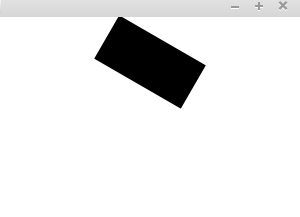## Moving the ship: First attempt

It's now possible to rotate the ship. Next, we'll add the possibility to move around. The ship should be able to move in any cardinal direction, which means that we need values for both x and y coordinates to represent movement. The concrete implementation of the movement is to modify the position of the polygon that represents the ship while the program is running.

Let's make use of the existing Point2D Java class to represent movement — the class has both x and y coordinates.

The first test version is to create a movement variable and adding it to the AnimationTimer class's handle method.

``Point2D movement = new Point2D(1, 0);``
``````new AnimationTimer() {

@Override
public void handle(long now) {
if(pressedKeys.getOrDefault(KeyCode.LEFT, false)) {
ship.setRotate(ship.getRotate() - 5);
}

if(pressedKeys.getOrDefault(KeyCode.RIGHT, false)) {
ship.setRotate(ship.getRotate() + 5);
}

ship.setTranslateX(ship.getTranslateX() + movement.getX());
}
}.start();``````

Hurray! The ship is moving (and it can be rotated). Although it disappears quite quickly...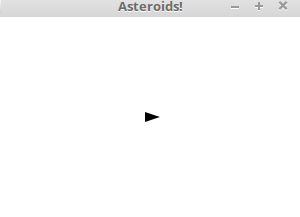The class that we chose, Point2D, is like the String class in some regards — namely, it is immutable so it cannot be modified. We cannot change the values of an existing point, and calling the methods of a point always returns a new point value. This poses something of a problem, since we cannot set the values of the objects to something else inside methods. The following solution is therefore ruled out.

``````new AnimationTimer() {

@Override
public void handle(long now) {
// .. does not work ..
if(pressedKeys.getOrDefault(KeyCode.UP, false)) {
}
// ..
}
}.start();``````

However, method calls are allowed. Looks like it's time for refactoring and clearing up the structure of the program...

## Moving the ship: Refactoring

Let's create a class called Ship, which contains a Polygon object and a Point2D object. The Polygon object represents the ship, and the Point2D object represents the movement of the ship. The ship receives the x and y coordinates of the ship as its constructor parameters. The ship can be rotated left or right.

``````import javafx.geometry.Point2D;
import javafx.scene.shape.Polygon;

public class Ship {

private Polygon character;
private Point2D movement;

public Ship(int x, int y) {
this.character = new Polygon(-5, -5, 10, 0, -5, 5);
this.character.setTranslateX(x);
this.character.setTranslateY(y);

this.movement = new Point2D(0, 0);
}

public Polygon getCharacter() {
return character;
}

public void turnLeft() {
this.character.setRotate(this.character.getRotate() - 5);
}

public void turnRight() {
this.character.setRotate(this.character.getRotate() + 5);
}

public void move() {
this.character.setTranslateX(this.character.getTranslateX() + this.movement.getX());
this.character.setTranslateY(this.character.getTranslateY() + this.movement.getY());
}
}``````

This refactoring leads to changes in the program in a couple of places. Instead of the point to represent movement and a polygon to represent the ship, we will create a Ship. In addition, the Pane object is given the Polygon object of the ship, but not the Ship object itself.

``````Ship ship = new Ship(150, 100);

The method in the AnimationTimer object should also be updated to use the methods of the ship.

``````new AnimationTimer() {

@Override
public void handle(long now) {
if(pressedKeys.getOrDefault(KeyCode.LEFT, false)) {
ship.turnLeft();
}

if(pressedKeys.getOrDefault(KeyCode.RIGHT, false)) {
ship.turnRight();
}

ship.move();
}

}.start();``````

## Moving the ship: Second attempt

The ship moves, but it's not possible to affect the movement yet. Let's add an acceleration functionality to the shpi. The ship should accelerate so that the speed accelerates to the direction that the ship points to. We can get the acceleration information from the rotation degree, which we can use the `getRotate()` method. We have already become well acquainted with it in rotating the ship.

The direction of the acceleration can be figured out with sine and cosine functions. The existing Java Math class contains the relevant methods. The methods assume their parameters to be in radians, so we are also going to need the Math class method that converts degrees into radians.

``````double changeX = Math.cos(Math.toRadians(*angle in degrees*));
double changeY = Math.sin(Math.toRadians(*angle in degrees*));``````

The first version of the accelerate method of the Ship class looks like this.

``````public void accelerate() {

}``````

Let's add the possibility to accelerate into the application. The accelerate method is called when the user is pressing the up button.

``````new AnimationTimer() {

@Override
public void handle(long now) {
if(pressedKeys.getOrDefault(KeyCode.LEFT, false)) {
ship.turnLeft();
}

if(pressedKeys.getOrDefault(KeyCode.RIGHT, false)) {
ship.turnRight();
}

if(pressedKeys.getOrDefault(KeyCode.UP, false)) {
ship.accelerate();
}

ship.move();
}
}.start();``````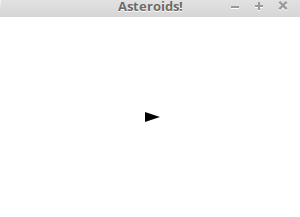As can be seen, the ship accelerates, indeed. The amount of acceleration is quite high, so it should be tweaked somewhat. Let's modify the accelerate mehtod of the ship so that the change is only 5% of the previous value.

``````public void accelerate() {

changeX *= 0.05;
changeY *= 0.05;

}``````

Now it's more or less possible to steer the ship.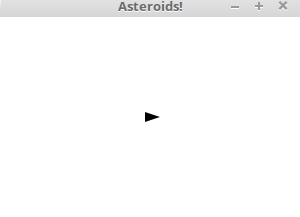## Creating an asteroid

Next up, we are going to create an asteroid. An asteroid has a shape, position, and movement.

Hmm..

Come to think of it, these are almost exactly the same things that a ship needs — the only difference is in the shape. This is a good moment to generalize. We'll create an abstract class called Character, which receives a polygon and position as its constructor parameters. Notice that the functionality is almost entirely copied from the class `Ship`.

``````import javafx.geometry.Point2D;
import javafx.scene.shape.Polygon;

public abstract class Character {

private Polygon character;
private Point2D movement;

public Character(Polygon polygon, int x, int y) {
this.character = polygon;
this.character.setTranslateX(x);
this.character.setTranslateY(y);

this.movement = new Point2D(0, 0);
}

public Polygon getCharacter() {
return character;
}

public void turnLeft() {
this.character.setRotate(this.character.getRotate() - 5);
}

public void turnRight() {
this.character.setRotate(this.character.getRotate() + 5);
}

public void move() {
this.character.setTranslateX(this.character.getTranslateX() + this.movement.getX());
this.character.setTranslateY(this.character.getTranslateY() + this.movement.getY());
}

public void accelerate() {

changeX *= 0.05;
changeY *= 0.05;

}
}``````

Then let's change the class Ship to inherit from the Character class.

``````import javafx.scene.shape.Polygon;

public class Ship extends Character {

public Ship(int x, int y) {
super(new Polygon(-5, -5, 10, 0, -5, 5), x, y);
}
}``````

Pretty straighforward stuff.

Then let's create the Asteroid class. The first draft is going to be a rectangle — we'll return to worry about the shape of the asteroid at a later stage.

``````import javafx.scene.shape.Polygon;

public class Asteroid extends Character {

public Asteroid(int x, int y) {
super(new Polygon(20, -20, 20, 20, -20, 20, -20, -20), x, y);
}
}``````
:

Then let's make sure that you can also add an asteroid to the application.

``````Pane pane = new Pane();
// setting the size ..

Ship ship = new Ship(150, 100);
Asteroid asteroid = new Asteroid(50, 50);

asteroid.turnRight();
asteroid.turnRight();
asteroid.accelerate();
asteroid.accelerate();``````

In order for an asteroid to move, the related move method must be called in the animation.

``````new AnimationTimer() {

@Override
public void handle(long now) {
if (pressedKeys.getOrDefault(KeyCode.LEFT, false)) {
ship.turnLeft();
}

if (pressedKeys.getOrDefault(KeyCode.RIGHT, false)) {
ship.turnRight();
}

if (pressedKeys.getOrDefault(KeyCode.UP, false)) {
ship.accelerate();
}

ship.move();
asteroid.move();
}
}.start();``````

Now the application contains both a ship and an asteroid.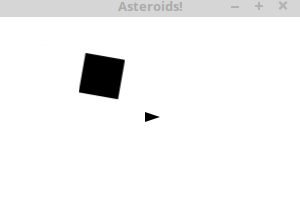## The collision between the ship and an asteroid

Next we will implement collision between a ship and an asteroid. If a ship collides with an asteroid, the `stop` method of an AnimationTimer -object is called, and the animation stops.

Both a ship and an asteroid are characters. Add a method for checking if two characters collide to the `Character` class. For now two characters never collide.

``````public boolean collide(Character other) {
return false;
}``````

The Shape class, which the Polygon class inherits, has a handy method for checking for collision. The method public static Shape intersect(Shape shape1, Shape shape2) returns the intersection of two Shape objects.

If the intersection is zero, there is no collision.

Let's modify the collide method so, that it uses the intersect method.

``````public boolean collide(Character other) {
Shape collisionArea = Shape.intersect(this.character, other.getCharacter());
return collisionArea.getBoundsInLocal().getWidth() != -1;
}``````

Let's also add functionality that stops the application if a collision happens.

``````new AnimationTimer() {

@Override
public void handle(long now) {
if (pressedKeys.getOrDefault(KeyCode.LEFT, false)) {
ship.turnLeft();
}

if (pressedKeys.getOrDefault(KeyCode.RIGHT, false)) {
ship.turnRight();
}

if (pressedKeys.getOrDefault(KeyCode.UP, false)) {
ship.accelerate();
}

ship.move();
asteroid.move();

if (ship.collide(asteroid)) {
stop();
}
}
}.start();``````

Now the application stops if a ship and an asteroid collide.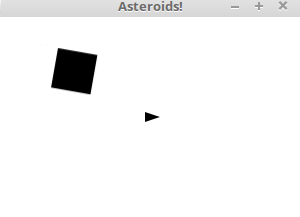## Multiple asteroids

Now we will add more asteroids. We can represent the asteroids as a list. In the example below we first create a ship and then add five asteroids.

``````Ship ship = new Ship(150, 100);
List<Asteroid> asteroids = new ArrayList<>();
for (int i = 0; i < 5; i++) {
Random rnd = new Random();
Asteroid asteroid = new Asteroid(rnd.nextInt(100), rnd.nextInt(100));
}

Let's modify drawing asteroids and checking for collision so that they work with multiple asteroids.

``````new AnimationTimer() {

@Override
public void handle(long now) {
if (pressedKeys.getOrDefault(KeyCode.LEFT, false)) {
ship.turnLeft();
}

if (pressedKeys.getOrDefault(KeyCode.RIGHT, false)) {
ship.turnRight();
}

if (pressedKeys.getOrDefault(KeyCode.UP, false)) {
ship.accelerate();
}

ship.move();
asteroids.forEach(asteroid -> asteroid.move());

asteroids.forEach(asteroid -> {
if (ship.collide(asteroid)) {
stop();
}
});

}
}.start();``````

Now when the application starts we see multiple asteroids.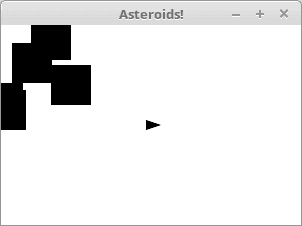At the moment every asteroid looks the same and moves the same way. It would be nice if there was some variance between the asteroids. Let's modify the Asteroid -class so, that it has a method for randomly assigning attributes to asteroids. We can decide that asteroids always have five corners, and their basic shape is always a pentagon. We can add variance to their shapes by moving the locations of the corners a little.

You can find the formula for calculating the angles of the corners of a pentagon from http://mathworld.wolfram.com/Pentagon.html. Below we have used the formula, and added some variance to the size of the asteroids and the locations of an asteroids corners.

``````import java.util.Random;
import javafx.scene.shape.Polygon;

public class PolygonFactory {

public Polygon createPolygon() {
Random rnd = new Random();

double size = 10 + rnd.nextInt(10);

Polygon polygon = new Polygon();
double c1 = Math.cos(Math.PI * 2 / 5);
double c2 = Math.cos(Math.PI / 5);
double s1 = Math.sin(Math.PI * 2 / 5);
double s2 = Math.sin(Math.PI * 4 / 5);

size, 0.0,
size * c1, -1 * size * s1,
-1 * size * c2, -1 * size * s2,
-1 * size * c2, size * s2,
size * c1, size * s1);

for (int i = 0; i < polygon.getPoints().size(); i++) {
int change = rnd.nextInt(5) - 2;
polygon.getPoints().set(i, polygon.getPoints().get(i) + change);
}

return polygon;
}
}``````

Let's modify the Asteroid class so that it uses the PolygonFactory class to create polygons.

``````public class Asteroid extends Character {

public Asteroid(int x, int y) {
super(new PolygonFactory().createPolygon(), x, y);
}

}``````

Now the asteroids look a bit more varied.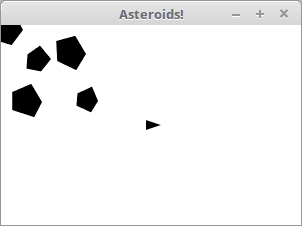We will also add movement and direction to the asteroids. Movement and direction have been partially defined in the Character class, but we want to add some randomness to the movement. When an asteroid is created, it's direction should be a random number between [0, 360]. Asteroids also move a little — the movement is defined as a random number of acceleration calls when the character is created. Finally an asteroid also rotates. Each time an asteroid moves, it also rotates a little.

``````import java.util.Random;

public class Asteroid extends Character {

private double rotationalMovement;

public Asteroid(int x, int y) {
super(new PolygonFactory().createPolygon(), x, y);

Random rnd = new Random();

super.getCharacter().setRotate(rnd.nextInt(360));

int accelerationAmount = 1 + rnd.nextInt(10);
for (int i = 0; i < accelerationAmount; i++) {
accelerate();
}

this.rotationalMovement = 0.5 - rnd.nextDouble();
}

@Override
public void move() {
super.move();
super.getCharacter().setRotate(super.getCharacter().getRotate() + rotationalMovement);
}
}``````

In the example below we use inheritance in the `move` method. When the move method is called, it first calls the move method from the Character class. Then the character is rotated. The final product is an asteroid with some rotational movement.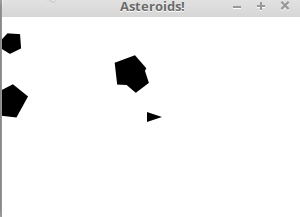## Staying within the window

The application is a bit boring, because the asteroids and the ship can leave the screen. Let's modify it so, that the characters stay on screen.

We will define constants width and height for the AsteroidsApplication. Each class can have class specific values using the keyword `static`. Below we define variables `WIDTH` and `HEIGHT`, which can be referenced elsewhere in the program code.

``````public class AsteroidsApplication extends Application {

public static int WIDTH = 300;
public static int HEIGHT = 200;

@Override
public void start(Stage stage) throws Exception {
Pane pane = new Pane();
pane.setPrefSize(WIDTH, HEIGHT);

Ship ship = new Ship(WIDTH / 2, HEIGHT / 2);
List<Asteroid> asteroids = new ArrayList<>();
for (int i = 0; i < 5; i++) {
Random rnd = new Random();
Asteroid asteroid = new Asteroid(rnd.nextInt(WIDTH / 3), rnd.nextInt(HEIGHT));
}

// ...``````

Variables with the keyword `static` are not part of objects made from the class. If a `static` variable is also `public` —like above — the variable can be referenced by other classes. Let's modify the move method of the Character class so that it uses the static variables of the AsteroidApplication, the class variables WIDTH and HEIGHT. The move method below checks that the character stays on screen.

``````public void move() {
this.character.setTranslateX(this.character.getTranslateX() + this.movement.getX());
this.character.setTranslateY(this.character.getTranslateY() + this.movement.getY());

if (this.character.getTranslateX() < 0) {
this.character.setTranslateX(this.character.getTranslateX() + AsteroidsApplication.LEVEYS);
}

if (this.character.getTranslateX() > AsteroidsApplication.LEVEYS) {
this.character.setTranslateX(this.character.getTranslateX() % AsteroidsApplication.LEVEYS);
}

if (this.character.getTranslateY() < 0) {
this.character.setTranslateY(this.character.getTranslateY() + AsteroidsApplication.KORKEUS);
}

if (this.character.getTranslateY() > AsteroidsApplication.KORKEUS) {
this.character.setTranslateY(this.character.getTranslateY() % AsteroidsApplication.KORKEUS);
}
}``````

Now the characters stay on screen.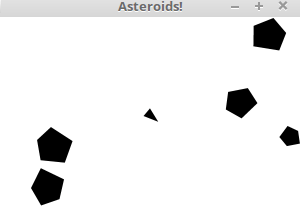We are not quite satisfied with this version of the application, because characters sometimes "jump" from one side of the screen to the other. The size of the character is not taken into account, so its x- or y -coordinates can be outside of the screen, even if part of the character stays visible. We can —probably— solve this problem with the getBoundsInParent-method of the Node -class. However we will not go more into it here.

## Projectiles

Playing asteroids without projectiles would be just dodging asteroids. Next we will add projectiles. Projectiles have a shape, a direction and movement. We can use the Character class for creating projectiles. Let's create the first version of the Projectile-class. For now all projectiles are squares.

``````import javafx.scene.shape.Polygon;

public class Projectile extends Character {

public Projectile(int x, int y) {
super(new Polygon(2, -2, 2, 2, -2, 2, -2, -2), x, y);
}

}``````

Contrary to ships and asteroids, we don't want any projectiles on screen when the application starts. We will declare a list for projectiles, but leave it empty for now.

``List<Projectile> projectiles = new ArrayList<>();``

A projectile is created when the user presses the spacebar. When a projectile is created its direction is the same as the ship's direction. Let's make the first version of shooting projectiles.

``````if (pressedKeys.getOrDefault(KeyCode.SPACE, false)) {
// we shoot
Projectile projectile = new Projectile((int) ship.getCharacter().getTranslateX(), (int) ship.getCharacter().getTranslateY());
projectile.getCharacter().setRotate(ship.getCharacter().getRotate());

}``````

Now the ship shoots a projectile when the user presses the spacebar, but the projectiles do not move. The projectiles do not hit other characters either.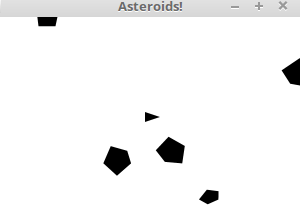We want to be able to modify the movement of a projectile. However, currently the `move` method of a Character is `private`, and we have to access to it through other methods. Let's add `getMovement` and `setMovement` to the `Character` class.

Now setting the speed of a projectile is straightforwards. We accelerate the speed of a projectile a bit (so it never stays put) and normalize the speed (in practice we handle the speed as a vector with the length of 1). Here the speed is multiplied by three.

``````if (pressedKeys.getOrDefault(KeyCode.SPACE, false)) {
// we shoot
Projectile projectile = new Projectile((int) ship.getCharacter().getTranslateX(), (int) ship.getCharacter().getTranslateY());
projectile.getCharacter().setRotate(ship.getCharacter().getRotate());

projectile.accelerate();
projectile.setMovement(projectile.getMovement().normalize().multiply(3));

}``````

Finally we add moving the projectiles to moving all other characters.

``````ship.move();
asteroids.forEach(asteroid -> asteroid.move());
projectiles.forEach(projectile -> projectile.move());``````

Now the projectiles move. They do not yet hit anything, and there are quite a lot of them... Let's limit the number of projectiles a bit — we can decide that there can only be 3 projectiles at once.

``````if (pressedKeys.getOrDefault(KeyCode.SPACE, false) && projectiles.size() < 3) {
// we shoot
Projectile projectile = new Projectile((int) ship.getCharacter().getTranslateX(), (int) ship.getCharacter().getTranslateY());
projectile.getCharacter().setRotate(ship.getCharacter().getRotate());

projectile.accelerate();
projectile.setMovement(projectile.getMovement().normalize().multiply(3));

}``````

Let's also add functionality for hitting asteroids. Projectiles can hit asteroids. If a projectile hits an asteroid, the asteroid is removed from the asteroid list and is not drawn.

``````projectiles.forEach(projectile -> {
List<Asteroid> collisions = asteroids.stream()
.filter(asteroid -> asteroid.collide(projectile))
.collect(Collectors.toList());

collisions.stream().forEach(collided -> {
asteroids.remove(collided);
pane.getChildren().remove(collided.getCharacter());
});
});``````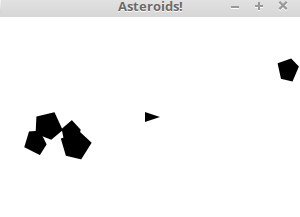The projectiles do not however disappear when they hit an asteroid. One way to remove the projectiles after a hit is described below.

``````List<Projectile> projectilesToRemove = projectiles.stream().filter(projectile -> {
List<Asteroidi> collisions = asteroids.stream()
.filter(asteroid -> asteroid.collide(projectile))
.collect(Collectors.toList());

if(collisions.isEmpty()) {
return false;
}

collisions.stream().forEach(collided -> {
asteroids.remove(collided);
pane.getChildren().remove(collided.getCharacter());
});

return true;
}).collect(Collectors.toList());

projectilesToRemove.forEach(projectile -> {
pane.getChildren().remove(projectile.getCharacter());
projectiles.remove(projectile);
});``````

It works, but we can improve it a bit. In practice this is declaring wether a Character is "in the game" or not. We could for example add an attribute "alive", which we could use to make things clearer. Using this attribute the code improves a bit.

``````projectiles.forEach(projectile -> {
asteroids.forEach(asteroid -> {
if(projectile.collide(asteroid)) {
projectile.setAlive(false);
asteroid.setAlive(false);
}
});
});

projectiles.stream()
.filter(projectile -> !projectile.isAlive())
.forEach(projectile -> pane.getChildren().remove(projectile.getCharacter()));
projectiles.removeAll(projectiles.stream()
.filter(projectile -> !projectile.isAlive())
.collect(Collectors.toList()));

asteroids.stream()
.filter(asteroid -> !asteroid.isAlive())
.forEach(asteroid -> pane.getChildren().remove(asteroid.getCharacter()));
asteroids.removeAll(asteroids.stream()
.filter(asteroid -> !asteroid.isAlive())
.collect(Collectors.toList()));``````

The lines at the end are almost identical — both are handling characters. Maybe we could refactor this a bit.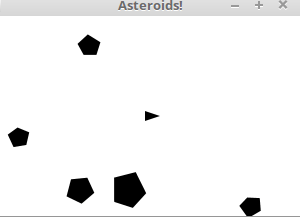Asteroids games almost always have a some sort of a points system. The points are displayed as text-objects, value of which changes when the number of points changes. We can decide that a player gets 1000 points every time they destroy an asteroid.

The Java Text -class is great for this purpose. A Text object has coordinates and content. In the example below the player always has 0 points.

``````@Override
public void start(Stage stage) throws Exception {
Pane pane = new Pane();
Text text = new Text(10, 20, "Points: 0");

Scene scene = new Scene(pane);
stage.setTitle("Asteroids!");
stage.setScene(scene);
stage.show();
}``````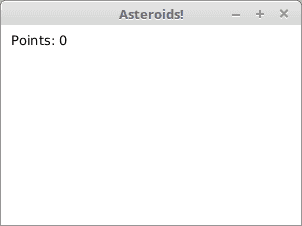However we want to be able to increase the number of points. One handy tool for this is the AtomicInteger-class, which offers integers as encapsulated objects. AtomicInteger also enables us to increase the points when a method is called.

``````@Override
public void start(Stage stage) throws Exception {
Pane pane = new Pane();
Text text = new Text(10, 20, "Points: 0");

AtomicInteger points = new AtomicInteger();

Scene scene = new Scene(pane);
stage.setTitle("Asteroids!");
stage.setScene(scene);
stage.show();

new AnimationTimer() {

@Override
public void handle(long now) {
text.setText("Points: " + points.incrementAndGet());
}
}.start();
}``````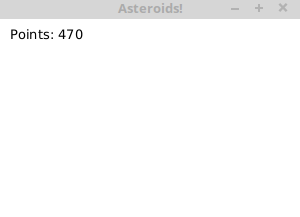Now we can display the number of points and increase it. Let's connect the points to the game so, that the number of points increases every time player's projectile hits an asteroid.

This can be done as a part of collision between a projectile and an asteroid.

``````projectiles.forEach(projectile -> {
asteroids.forEach(asteroid -> {
if(projectile.collide(asteroid)) {
projectile.setAlive(false);
asteroid.setAlive(false);
}
});

if(!projectile.isAlive()) {
}
});``````

Now, if the increasing of points has been removed from the start of the animation timer, player gets points when they hit an asteroid.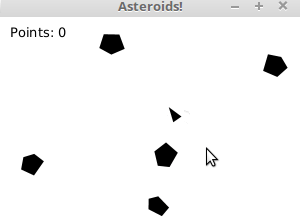When we hit an asteroid, they disappear, and soon there is nothing to shoot. This is not acceptable!

We will add a function which adds asteroids throughout the game. A new asteroid is added with the probability of 0.5% each time the AnimationTimer-object is called. A new asteroid is added only if it does not collide with a ship immediately.

The handle method of an AnimationTimer-object is called approximately 60 times a second, so in ten seconds a few asteroids are added. We add the call to the end of the handle -method.

``````if(Math.random() < 0.005) {
Asteroid asteroid = new Asteroid(WIDTH, HEIGHT);
if(!asteroid.collide(ship)) {